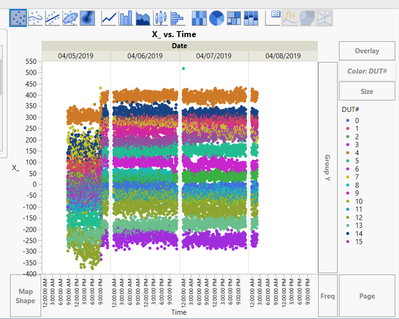Choose Language Hide Translation Bar
Highlighted

## Data Manipulation - How do I remove Initial Offsets specific to a col identifier

Hi all,

My first post! I have a regular set of data I process and have been doing manual manipulation in excel before importing and plotting in JMP. I'm sure someone has a better way :)

I measure 16 DUT's and record offset data for each dut. I would like to zero out each individual DUT offset based on the first 100samples of data and apply this to all rows of data.

I would like to remove the initial offset based of "X_" on average of say the first 100 rows. This offset is unique to each DUT identified in COl "DUT#".

Is there a slick way of doing this via formula or built in scripting options?

My manual method is to split table by DUT, create 16 new cols, subtract an average of the first 100 data and then restack it.

I have attached the plot and .jmp table showing raw data plotted without the intiial offset being removed.Ultimately I want to see the relative change over time hence removal of the intiial offset!

One other challenge is in dealing with the Date / Time cols. Note I plotted Time / Date, ideally I would like to calculate the time in hours starting from zero to the end of dataset and plot from zero to say 85hours on the X Axis of this plot. I'm struggling to manipulate this using the formula!

Any help appreciated to make my day more productive!!

Regards,

Mark

1 ACCEPTED SOLUTION

Accepted Solutions
Highlighted

## Re: Data Manipulation - How do I remove Initial Offsets specific to a col identifier

Mark,

Here is a short script that should take care of the offsets.  Attached is a version of you file that shows the column formulas.  Note that I also created a "Date Time" column.

``````dt = Current Data Table();
dtStart = dt << subset( rows( 1 :: 160 ), all columns( 1 ) );
Summarize( dtStart, DUT = by( :name( "DUT#" ) ), startMeans = Mean( :X_ ) );
offsets = Associative Array( DUT, startMeans );
Close( dtStart, nosave );
For( i = 1, i <= N Rows( dt ), i++,
Column( dt, "X_NORM" )[i] = Column( dt, "X_" )[i] - offsets[Char( Column( dt, "DUT#" )[i] )]
);``````

2 REPLIES 2
Highlighted

## Re: Data Manipulation - How do I remove Initial Offsets specific to a col identifier

Mark,

Here is a short script that should take care of the offsets.  Attached is a version of you file that shows the column formulas.  Note that I also created a "Date Time" column.

``````dt = Current Data Table();
dtStart = dt << subset( rows( 1 :: 160 ), all columns( 1 ) );
Summarize( dtStart, DUT = by( :name( "DUT#" ) ), startMeans = Mean( :X_ ) );
offsets = Associative Array( DUT, startMeans );
Close( dtStart, nosave );
For( i = 1, i <= N Rows( dt ), i++,
Column( dt, "X_NORM" )[i] = Column( dt, "X_" )[i] - offsets[Char( Column( dt, "DUT#" )[i] )]
);``````

Highlighted

## Re: Data Manipulation - How do I remove Initial Offsets specific to a col identifier

Wow - thanks John! this saves an immense amount of time.

I'm not familiar with the functions you have used so I'm going to study them and figure out how to scale this across all the cols!

Mark

Article Labels

There are no labels assigned to this post.